##帳號 自動登錄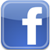找回密碼 密碼 註冊

# [教學] python時間序列預測的7種方法發表於 2021-7-12 22:39:03 | 顯示全部樓層 |閱讀模式時間序列預測在日常分析中常會用到，前段時間在處理預算相關的內容，涉到一些指標預測，學習到了這篇文章，整理出來分享給大家。 目錄         數據準備 方法1：樸素法 方法2：簡單平均法 方法3：移動平均法 方法4：簡單指數平滑法 方法5：霍爾特(Holt)線性趨勢法 方法6：Holt-Winters季節性預測模型 方法7：自回歸移動平均模型（ARIMA） 總結 相關文章: 數據準備 數據集（JetRail高鐵的乘客數量）下載，鏈接: https://github.com/thao9611/Time_Series_Analysis 假設要解決一個時序問題：根據過往兩年的數據（2012 年8 月至2014 年8月），需要用這些數據預測接下來7 個月的乘客數量。 import pandas as pd import numpy as np import matplotlib.pyplot as plt df = pd. read_csv ( 'train.csv' ) df. head ()複製代碼 python,時間序列,預測依照上面的代碼，我們獲得了2012-2014 年兩年每個小時的乘客數量。為了解釋每種方法的不同之處，以每天為單位構造和聚合了一個數據集。 從2012 年8 月- 2013 年12 月的數據中構造一個數據集。 創建train and test 文件用於建模。前14 個月（ 2012 年8 月- 2013 年10 月）用作訓練數據，後兩個月（2013 年11 月– 2013 年12 月）用作測試數據。 以每天為單位聚合數據集。 import pandas as pd import matplotlib.pyplot as plt # Subsetting the dataset # Index 11856 marks the end of year 2013 df = pd.read_csv('train.csv', nrows=11856) # Creating train and test set # Index 10392 marks the end of October 2013 train = df[0:10392] test = df[10392:14000] # Aggregating the dataset at daily level df['Timestamp'] = pd.to_datetime(df['Datetime'], format='%d-%m-%Y %H:%M') df.index = df['Timestamp'] df = df.resample('D').mean() train['Timestamp'] = pd.to_datetime(train['Datetime'], format='%d-%m-%Y %H:%M') train.index = train['Timestamp'] train = train.resample('D').mean() test['Timestamp'] = pd.to_datetime(test['Datetime'], format='%d-%m-%Y %H:%M') test.index = test['Timestamp'] test = test.resample('D').mean() #Plotting data train.Count.plot(figsize=(15,8), title= 'Daily Ridership', fontsize=14) test.Count.plot(figsize=(15,8), title= 'Daily Ridership', fontsize=14) plt.show()複製代碼 我們將數據可視化（訓練數據和測試數據一起），從而得知在一段時間內數據是如何變化的。 python,時間序列,預測方法1：樸素法 假設y 軸表示物品的價格，x 軸表示時間（天）。 python,時間序列,預測如果數據集在一段時間內都很穩定，我們想預測第二天的價格，可以取前面一天的價格，預測第二天的值。這種假設第一個預測點和上一個觀察點相等的預測方法就叫樸素法。 python,時間序列,預測dd = np.asarray(train['Count']) y_hat = test.copy() y_hat['naive'] = dd[len(dd) - 1] plt.figure(figsize=(12, 8)) plt.plot(train.index, train['Count'], label='Train') plt.plot(test.index, test['Count'], label='Test') plt.plot(y_hat.index, y_hat['naive'], label='Naive Forecast') plt.legend(loc='best') plt.title("Naive Forecast") plt.show()複製代碼 python,時間序列,預測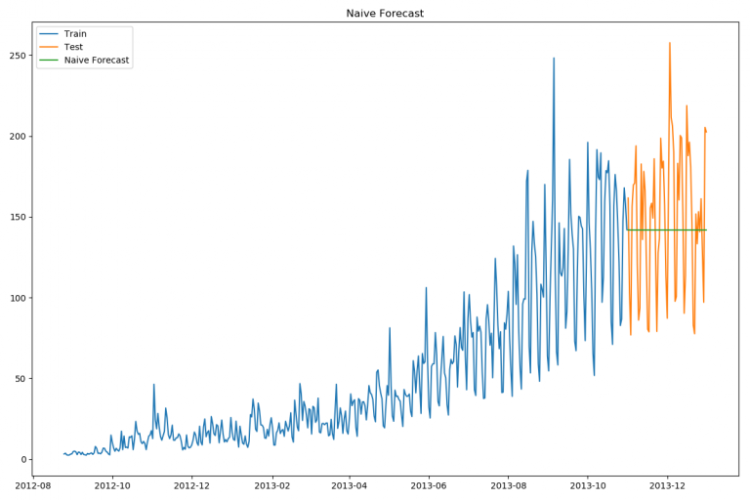樸素法並不適合變化很大的數據集，最適合穩定性很高的數據集。我們計算下均方根誤差，檢查模型在測試數據集上的準確率： from sklearn.metrics import mean_squared_error from math import sqrt rms = sqrt(mean_squared_error(test['Count'], y_hat['naive'])) print(rms)複製代碼 最終均方根誤差RMS為：43.91640614391676 方法2：簡單平均法 我們假設y軸表示某個物品的價格，x軸表示時間（天）。 python,時間序列,預測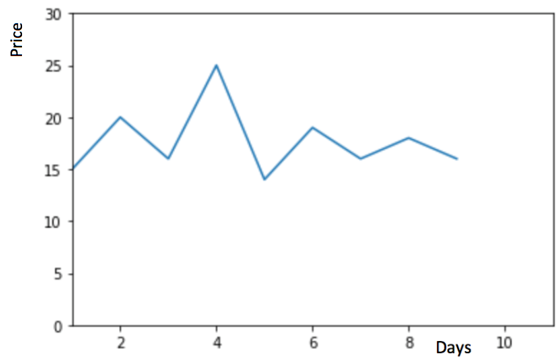物品價格會隨機上漲和下跌，平均價格會保持一致。我們經常會遇到一些數據集，雖然在一定時期內出現小幅變動，但每個時間段的平均值確實保持不變。這種情況下，我們可以預測出第二天的價格大致和過去天數的價格平均值一致。這種將預期值等同於之前所有觀測點的平均值的預測方法就叫簡單平均法。 python,時間序列,預測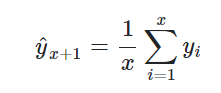y_hat_avg = test.copy() y_hat_avg['avg_forecast'] = train['Count'].mean() plt.figure(figsize=(12,8)) plt.plot(train['Count'], label='Train') plt.plot(test['Count'], label='Test') plt.plot(y_hat_avg['avg_forecast'], label='Average Forecast') plt.legend(loc='best') plt.show()複製代碼 python,時間序列,預測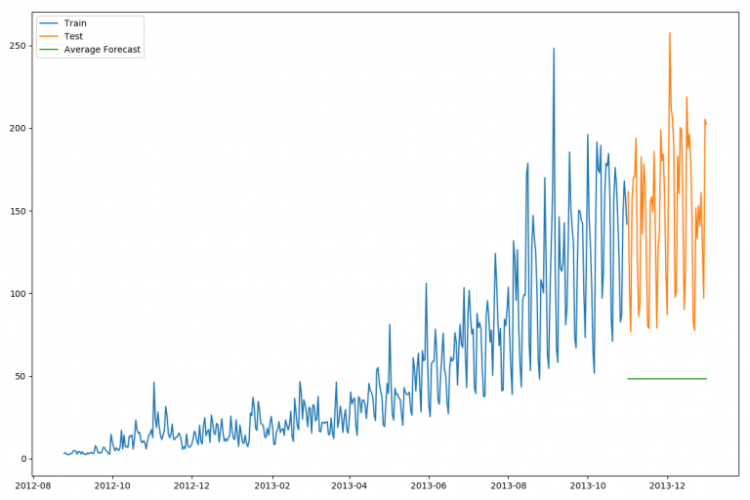用之前全部已知的值計算出它們的平均值，將它作為要預測的下一個值。當然這不會很準確，但這種預測方法在某些情況下效果是最好的。 該方法的均方根差為：109.88526527082863 from sklearn.metrics import mean_squared_error from math import sqrt rms = sqrt(mean_squared_error(test['Count'], y_hat_avg['avg_forecast'])) print(rms)複製代碼 這種模型並沒有改善準確率。因此我們可以從中推斷出當每個時間段的平均值保持不變時，這種方法的效果才能達到最好。雖然樸素法的準確率高於簡單平均法，但這並不意味著樸素法在所有的數據集上都比簡單平均法好。 方法3：移動平均法 假設y軸表示某個物品的價格，x軸表示時間（天）。 python,時間序列,預測物品價格在一段時間內大幅上漲，但後來又趨於平穩。我們也經常會遇到這種數據集，比如價格或銷售額某段時間大幅上升或下降。如果我們這時用之前的簡單平均法，就得使用所有先前數據的平均值，但在這裡使用之前的所有數據是說不通的，因為用開始階段的價格值會大幅影響接下來日期的預測值。因此，我們只取最近幾個時期的價格平均值。很明顯這裡的邏輯是只有最近的值最要緊。這種用某些窗口期計算平均值的預測方法就叫移動平均法。 計算移動平均值涉及到一個有時被稱為“滑動窗口”的大小值p。使用簡單的移動平均模型，我們可以根據之前數值的固定有限數p的平均值預測某個時序中的下一個值。這樣，對於所有的i > p： python,時間序列,預測移動平均法實際上很有效，特別是當你為時序選擇了正確的p值時。（以下程序選擇了60天作為窗口大小） y_hat_avg = test.copy() y_hat_avg['moving_avg_forecast'] = train['Count'].rolling(60).mean().iloc[-1] plt.figure(figsize=(16,8)) plt.plot(train['Count'], label='Train') plt.plot(test['Count'], label='Test') plt.plot(y_hat_avg['moving_avg_forecast'], label='Moving Average Forecast') plt.legend(loc='best') plt.show()複製代碼 python,時間序列,預測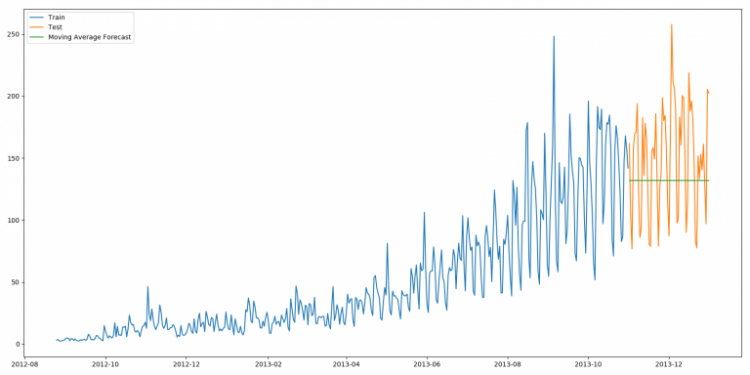此方法計算出來的均方根差為：46.72840725106963 from sklearn.metrics import mean_squared_error from math import sqrt rms = sqrt(mean_squared_error(test['Count'], y_hat_avg['moving_avg_forecast'])) print(rms)複製代碼 可以看到，對於這個數據集，樸素法比簡單平均法和移動平均法的表現要好。此外，我們還可以試試簡單指數平滑法，它比移動平均法的一個進步之處就是相當於對移動平均法進行了加權。在上文移動平均法可以看到，我們對“p”中的觀察值賦予了同樣的權重。但是我們可能遇到一些情況，比如“p”中每個觀察值會以不同的方式影響預測結果。將過去觀察值賦予不同權重的方法就叫做加權移動平均法。加權移動平均法其實還是一種移動平均法，只是“滑動窗口期”內的值被賦予不同的權重，通常來講，最近時間點的值發揮的作用更大了。 python,時間序列,預測這種方法並非選擇一個窗口期的值，而是需要一列權重值（相加後為1）。例如，如果我們選擇[0.40, 0.25, 0.20, 0.15]作為權值，我們會為最近的4個時間點分別賦給40%，25%，20%和15%的權重。 方法4：簡單指數平滑法 我們注意到簡單平均法和加權移動平均法在選取時間點的思路上存在較大的差異。我們就需要在這兩種方法之間取一個折中的方法，在將所有數據考慮在內的同時也能給數據賦予不同非權重。例如，相比更早時期內的觀測值，它會給近期的觀測值賦予更大的權重。按照這種原則工作的方法就叫做簡單指數平滑法。它通過加權平均值計算出預測值，其中權重隨著觀測值從早期到晚期的變化呈指數級下降，最小的權重和最早的觀測值相關： python,時間序列,預測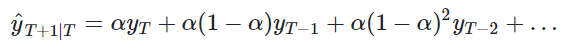其中0≤α≤1是平滑參數。對時間點T+1的單步預測值是時序的所有觀測值的加權平均數。權重下降的速率由參數α控制，預測值是與的和。 因此，它可以寫為： python,時間序列,預測所以本質上，我們是用兩個權重α和1−α得到一個加權移動平均值。我們可以看到和1−α相乘，讓表達式呈遞進形式，這也是該方法被稱為“指數”的原因。時間t+1 處的預測值為最近觀測值和最近預測值 之間的加權平均值。 from statsmodels.tsa.api import SimpleExpSmoothing y_hat_avg = test.copy() fit = SimpleExpSmoothing(np.asarray(train['Count'])).fit(smoothing_level=0.6, optimized=False) y_hat_avg['SES'] = fit.forecast(len(test)) plt.figure(figsize=(16, 8)) plt.plot(train['Count'], label='Train') plt.plot(test['Count'], label='Test') plt.plot(y_hat_avg['SES'], label='SES') plt.legend(loc='best') plt.show()複製代碼 上述方法計算出來的均方根差為：43.357625225228155 from sklearn.metrics import mean_squared_error from math import sqrt rms = sqrt(mean_squared_error(test['Count'], y_hat_avg['SES'])) print(rms)複製代碼 模型中使用的α值為0.6，我們可以用測試集繼續調整參數以生成一個更好的模型。 方法5：霍爾特(Holt)線性趨勢法 假設y軸表示某個物品的價格，x軸表示時間（天）。 python,時間序列,預測如果物品的價格是不斷上漲的（見上圖），我們上面的方法並沒有考慮這種趨勢，即我們在一段時間內觀察到的價格的總體模式。在上圖例子中，我們可以看到物品的價格呈上漲趨勢。雖然上面這些方法都可以應用於這種趨勢，但我們仍需要一種方法可以在無需假設的情況下，準確預測出價格趨勢。這種考慮到數據集變化趨勢的方法就叫做霍爾特線性趨勢法。 每個時序數據集可以分解為相應的幾個部分：趨勢（Trend），季節性(Seasonal)和殘差(Residual)。任何呈現某種趨勢的數據集都可以用霍爾特線性趨勢法用於預測。 import statsmodels.api as sm sm.tsa.seasonal_decompose(train['Count']).plot() result = sm.tsa.stattools.adfuller(train['Count']) plt.show()複製代碼 python,時間序列,預測我們從圖中可以看出，該數據集呈上升趨勢。因此我們可以用霍爾特線性趨勢法預測未來價格。該算法包含三個方程：一個水平方程，一個趨勢方程，一個方程將二者相加以得到預測值y^： python,時間序列,預測我們在上面算法中預測的值稱為水平（level）。正如簡單指數平滑一樣，這裡的水平方程顯示它是觀測值和样本內單步預測值的加權平均數，趨勢方程顯示它是根據ℓ(t)−ℓ(t−1) 和之前的預測趨勢b (t−1) 在時間t處的預測趨勢的加權平均值。 我們將這兩個方程相加，得出一個預測函數。我們也可以將兩者相乘而不是相加得到一個乘法預測方程。當趨勢呈線性增加和下降時，我們用相加得到的方程；當趨勢呈指數級增加或下降時，我們用相乘得到的方程。實踐操作顯示，用相乘得到的方程，預測結果會更穩定，但用相加得到的方程，更容易理解。 python,時間序列,預測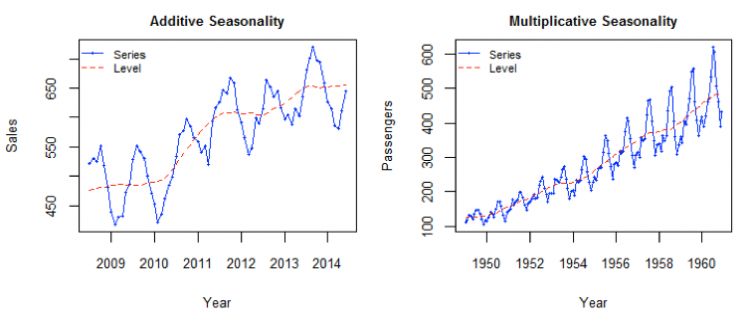from statsmodels.tsa.api import Holt y_hat_avg = test.copy() fit = Holt(np.asarray(train['Count'])).fit(smoothing_level=0.3, smoothing_slope=0.1) y_hat_avg['Holt_linear'] = fit.forecast(len(test)) plt.figure(figsize=(16, 8)) plt.plot(train['Count'], label='Train') plt.plot(test['Count'], label='Test') plt.plot(y_hat_avg['Holt_linear'], label='Holt_linear') plt.legend(loc='best') plt.show()複製代碼 python,時間序列,預測使用該方法的均方根誤差為：43.056259611507286 from sklearn.metrics import mean_squared_error from math import sqrt rms = sqrt(mean_squared_error(test['Count'], y_hat_avg['Holt_linear'])) print(rms)複製代碼 這種方法能夠準確地顯示出趨勢，因此比前面的幾種模型效果更好。如果調整一下參數，結果會更好。 方法6：Holt-Winters季節性預測模型 在應用這種算法前，我們先介紹一個新術語。假如有家酒店坐落在半山腰上，夏季的時候生意很好，顧客很多，但每年其餘時間顧客很少。因此，每年夏季的收入會遠高於其它季節，而且每年都是這樣，那麼這種重複現象叫做“季節性”（Seasonality）。如果數據集在一定時間段內的固定區間內呈現相似的模式，那麼該數據集就具有季節性。 python,時間序列,預測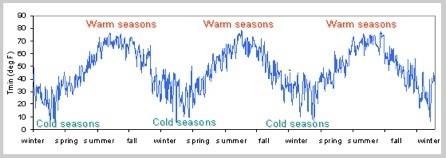我們之前討論的5種模型在預測時並沒有考慮到數據集的季節性，因此我們需要一種能考慮這種因素的方法。應用到這種情況下的算法就叫做Holt-Winters季節性預測模型，它是一種三次指數平滑預測，其背後的理念就是除了水平和趨勢外，還將指數平滑應用到季節分量上。 Holt-Winters季節性預測模型由預測函數和三次平滑函數——一個是水平函數，一個是趨勢函數，一個是季節分量 ，以及平滑參數α,β和γ。 python,時間序列,預測其中s 為季節循環的長度，0≤α≤ 1, 0 ≤β≤ 1 ， 0≤γ≤ 1。水平函數為季節性調整的觀測值和時間點t處非季節預測之間的加權平均值。趨勢函數和霍爾特線性方法中的含義相同。季節函數為當前季節指數和去年同一季節的季節性指數之間的加權平均值。在本算法，我們同樣可以用相加和相乘的方法。當季節性變化大致相同時，優先選擇相加方法，而當季節變化的幅度與各時間段的水平成正比時，優先選擇相乘的方法。 from statsmodels.tsa.api import ExponentialSmoothing y_hat_avg = test.copy() fit1 = ExponentialSmoothing(np.asarray(train['Count']), seasonal_periods=7, trend='add', seasonal='add', ).fit() y_hat_avg['Holt_Winter'] = fit1.forecast(len(test)) plt.figure(figsize=(16, 8)) plt.plot(train['Count'], label='Train') plt.plot(test['Count'], label='Test') plt.plot(y_hat_avg['Holt_Winter'], label='Holt_Winter') plt.legend(loc='best') plt.show()複製代碼 python,時間序列,預測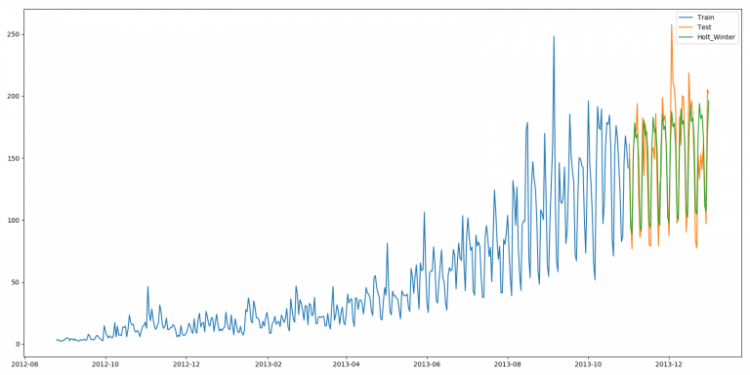使用該方法的均方根誤差為：23.961492566159794 from sklearn.metrics import mean_squared_error from math import sqrt rms = sqrt(mean_squared_error(test['Count'], y_hat_avg['Holt_Winter'])) print(rms)複製代碼 我們可以看到趨勢和季節性的預測準確度都很高。我們選擇了seasonal_period = 7作為每週重複的數據。也可以調整其它其它參數，我在搭建這個模型的時候用的是默認參數。你可以試著調整參數來優化模型。 方法7：自回歸移動平均模型（ARIMA） 另一個場景的時序模型是自回歸移動平均模型（ARIMA）。指數平滑模型都是基於數據中的趨勢和季節性的描述，而自回歸移動平均模型的目標是描述數據中彼此之間的關係。ARIMA的一個優化版就是季節性ARIMA。它像Holt-Winters季節性預測模型一樣，也把數據集的季節性考慮在內。 import statsmodels.api as sm y_hat_avg = test.copy() fit1 = sm.tsa.statespace.SARIMAX(train.Count, order=(2, 1, 4), seasonal_order=(0, 1, 1, 7)).fit() y_hat_avg['SARIMA'] = fit1.predict(start="2013-11-1", end="2013-12-31", dynamic=True) plt.figure(figsize=(16, 8)) plt.plot(train['Count'], label='Train') plt.plot(test['Count'], label='Test') plt.plot(y_hat_avg['SARIMA'], label='SARIMA') plt.legend(loc='best') plt.show()複製代碼 python,時間序列,預測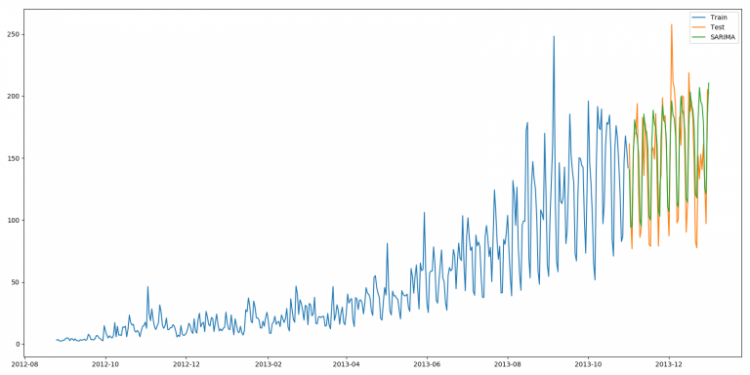使用該方法的均方根誤差為：26.052705330843708 from sklearn.metrics import mean_squared_error from math import sqrt rms = sqrt(mean_squared_error(test['Count'], y_hat_avg['SARIMA'])) print(rms)複製代碼 我們可以看到使用季節性ARIMA 的效果和Holt-Winters差不多。我們根據ACF（自相關函數）和PACF（偏自相關） 圖選擇參數。如果你為ARIMA 模型選擇參數時遇到了困難，可以用R 語言中的auto.arima。 總結 最後，我們將這幾種模型的準確度比較一下： python,時間序列,預測希望本文對你有所幫助，在解決時許問題的時候能從容以對。我建議你在解決問題時，可以依次試試這幾種模型，看看哪個效果最好。從上文也知道，數據集不同，每種模型的效果都有可能優於其它模型。因此，如果一個模型在某個數據集上效果很好，並不代表它在所有數據集上都比其它模型好。 原文地址：7 methods to perform Time Series forecasting (with Python codes) 文章出處

 本版積分規則 回帖後跳轉到最後一頁
﻿

GMT+8, 2021-7-24 10:05 , Processed in 0.068329 second(s), 24 queries .